Splitting a Table Full of Strings into Columns
Published: Jun 29, 2022
This was a fun afternoon I spent getting this working nicely. I don’t know if there are better ways of doing this, but if there are then the internet isn’t the place to look because if you type in string split you’ll get a whole bunch of pages about splitting a string… but just an individual string.

What I wanted was to be able to take a table full of delimited strings and make them into columns so that I could insert them into a table. So here’s what happened…

Firstly, it’ll be good to follow along with this one so if you have AdventureWorks (and SQL 2019, otherwise swap out the CONCAT_WS for something that works in your version) then you can create a set of delimited data as follows:

select top 1000 concat_ws('|', salesOrderID, SalesOrderNumber, AccountNumber, CustomerID, SalesPersonID, TotalDue)from AdventureWorks2012.Sales.SalesOrderHeaderwhere SalesPersonID is not nullgo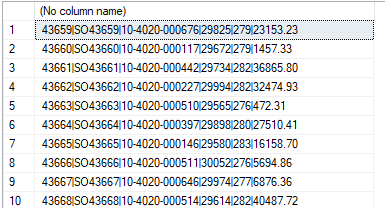Now what we’re going to do is pretend that this output is data we have received in a txt file from a client (because that’s what happened in my case).

Now, here’s where a huge caveat comes in… I COULD use SSIS to import this mystery txt file into SQL Server BUT this wasn’t the only file. Files came in by the dozen, all with differing numbers of columns etc. Therefore, I COULD have used SSIS, but the overhead in making a package which catered for every eventuality (and of which more variations could arise in future), was too onerous.

So I thought I’d give SQL Server a shot at it.

Let’s drop and re-create a table to store our new data:

drop table if exists importSalesgocreate table importSales(    salesRowID int identity(1, 1),    salesRaw varchar(1000))go

Now we can get going.

Firstly, we know that to split a string we can now (in SQL Server 2016+) use string_split.

select valuefrom string_split('43659|SO43659|10-4020-000676|29825|279|23153.23', '|')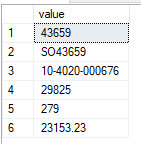This works great for a single string, but what about a whole table of strings?

select *from importSales icross apply string_split(salesRaw, '|') s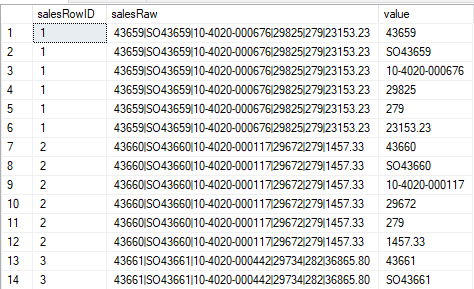Not a problem.

So what we can do now is add a partitioned row_number to the equation in order to effectively create a ColumnID on our data:

select row_number() over(partition by salesRowID order by salesRowID) columnID, i.salesRowID, i.salesRaw, s.valuefrom importSales icross apply string_split(salesRaw, '|') s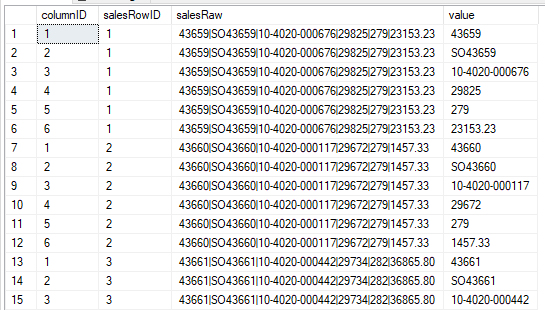We’ll put this into a temporary table in order to make this a little easier to work with going forwards:

drop table if exists #tempselect row_number() over(partition by salesRowID order by salesRowID) columnID, i.salesRowID, i.salesRaw, s.value into #tempfrom importSales icross apply string_split(salesRaw, '|') s

Now we’ve got a lot of rows, but we need this in a column format in order to place it into our table.

Conceptually, this is quite easy:

select *from #tempwhere columnID = 1select *from #tempwhere columnID = 2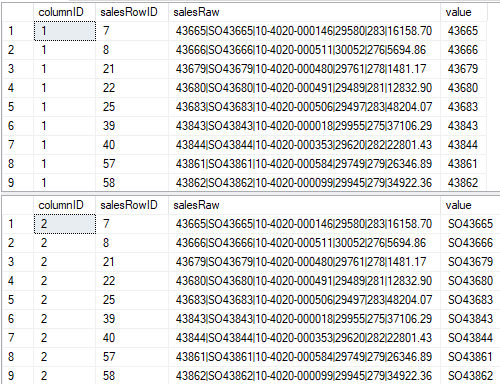We can now easily get each column’s data for each row. In the above we can join these two tables together on salesRowID and be done:

select t1.salesRowID, t1.salesRaw,t1.columnID, t1.value,t2.columnID, t2.valuefrom #temp t1join #temp t2on t1.salesRowID = t2.salesRowIDwhere t1.columnID = 1and t2.columnID = 2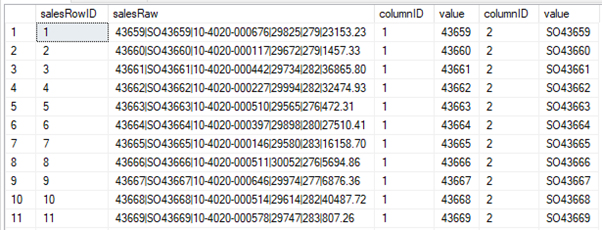Likewise to add in another column:

select t1.salesRowID, t1.salesRaw,t1.columnID, t1.value,t2.columnID, t2.value,t3.columnID, t3.valuefrom #temp t1join #temp t2on t1.salesRowID = t2.salesRowIDjoin #temp t3on t1.salesRowID = t3.salesRowIDwhere t1.columnID = 1and t2.columnID = 2and t3.columnID = 3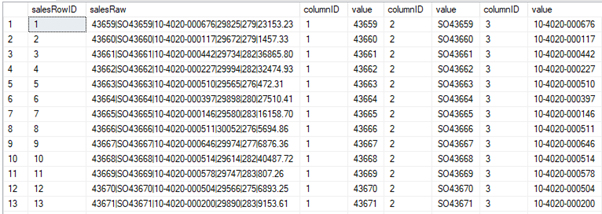Hopefully you’re seeing a pattern now?

Therefore why don’t we use some dynamic SQL in order to build that pattern out?

We need a starting point, a basis for our query to be built:

declare @select varchar(max), @tables varchar(max), @where varchar(max)select @select = 'select t1.value'select @tables = 'from #temp t1'select @where = 'where t1.columnID = 1'

Now that we have our starting point we know, based on the columnID, how many joins we need to make… it’s the same as the number of columns we have:

declare @numJoins int = (select max(columnID) from #temp)

We’re now going to write a WHILE loop in order to build our statement to the pattern we’ve already determined. You can see that here:

declare @joinCounter int = 2-- we know how many joins we need, so go add them...while @joinCounter <= @numJoinsbegin     select @select += (', t' + convert(varchar(10), @joinCounter) + '.value'),                -- we're using the pattern ', tX.value'                    @tables += ('join #temp t' + convert(varchar(10), @joinCounter) + 'on t1.salesRowID = t' + convert(varchar(10), @joinCounter) + '.salesRowID'),                -- using the pattern:-- join #temp tX-- on t1.salesRowID = tX.salesRowID@where += ('and t' + convert(varchar(10), @joinCounter) + '.columnID = ' + convert(varchar(10), @joinCounter))                -- using the pattern:-- and tX.columnID = Xfrom (select distinct columnID     from #temp     where columnID = @joinCounter) x                -- add the values for each column required    select @joinCounter += 1end

And if we look at the output of our PRINT statement you can see we were successful: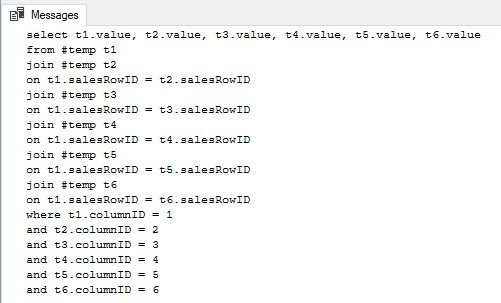So now let’s run the statement instead of just printing the results:

declare @numJoins int = (select max(columnID) from #temp)declare @select varchar(max), @tables varchar(max), @where varchar(max)select @select = 'select t1.value'select @tables = 'from #temp t1'select @where = 'where t1.columnID = 1'declare @joinCounter int = 2while @joinCounter <= @numJoinsbegin    select @select += (', t' + convert(varchar(10), @joinCounter) + '.value'),                @tables += ('join #temp t' + convert(varchar(10), @joinCounter) + 'on t1.salesRowID = t' + convert(varchar(10), @joinCounter) + '.salesRowID'),                @where += ('and t' + convert(varchar(10), @joinCounter)) + '.columnID = ' + convert(varchar(10), @joinCounter)    from (select distinct columnID from #temp where columnID = @joinCounter) x        select @joinCounter += 1enddeclare @sql varchar(max)select @sql = @select + '' + @tables + '' + @whereprint @sqlexec(@sql)go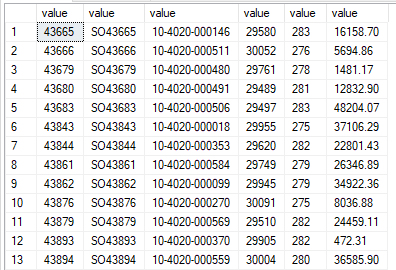We have now turned our delimited strings into a table. Exactly what we wanted.

So, how is this a solution? Well… imagine we’ve imported our data into some tables with standard names:

-- Make imports genericdrop table if exists testImport1, testImport2gocreate table testImport1(    importID int identity(1, 1),    importData varchar(1000))create table testImport2(    importID int identity(1, 1),    importData varchar(1000))goinsert into testImport1select top 50 concat_ws('|', salesOrderID, SalesOrderNumber, TotalDue)from AdventureWorks2012.Sales.SalesOrderHeaderinsert into testImport2select top 50 concat_ws('|', salesOrderID, AccountNumber, CustomerID, TerritoryID, CreditCardApprovalCode, TotalDue)from AdventureWorks2012.Sales.SalesOrderHeadergoselect *from testImport1select *from testImport2go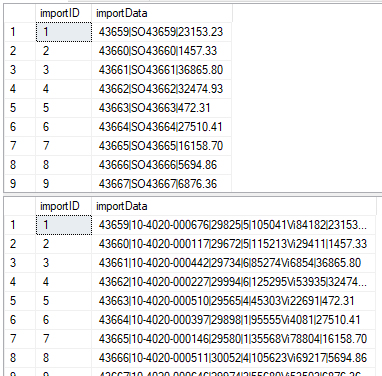You can see that we now have two tables with similar data (two columns with the same column names), yet completely different sized data and therefore different columnar outputs.

So what we’ll do now is to wrap our logic into a procedure:

-- Wrap into a procdrop procedure if exists columnSplitgocreate procedure columnSplit     @tableName varchar(250)as    create table #temp    (        columnID int,         importID int,         importData varchar(1000)    )        declare @table varchar(max)        select @table = 'select row_number() over(partition by importID order by importID) columnID, i.importID, s.valuefrom ' + @tableName + ' icross apply string_split(importData, ''|'') s'        insert into #temp     exec(@table)          declare @numJoins int = (select max(columnID) from #temp)          declare @select varchar(max), @tables varchar(max), @where varchar(max)          select @select = 'select t1.importData'     select @tables = 'from #temp t1'     select @where = 'where t1.columnID = 1'          declare @joinCounter int = 2          while @joinCounter <= @numJoins     begin         select @select += (', t' + convert(varchar(10), @joinCounter) + '.importData'),                     @tables += ('join #temp t' + convert(varchar(10), @joinCounter) + 'on t1.importID = t' + convert(varchar(10), @joinCounter) + '.importID'),                     @where += ('and t' + convert(varchar(10), @joinCounter)) + '.columnID = ' + convert(varchar(10), @joinCounter)         from (select distinct columnID from #temp where columnID = @joinCounter) x                  select @joinCounter += 1     end          declare @sql varchar(max)          select @sql = @select + '' + @tables + '' + @where          print @sql          exec(@sql)go

Once that’s done we can now simply call our procedure, passing in the name of each of our raw data tables, no matter what the data inside them or the resulting schema, and our procedure will turn them into tables for us. Awesome:

exec columnSplit 'testImport1'goexec columnSplit 'testImport2'go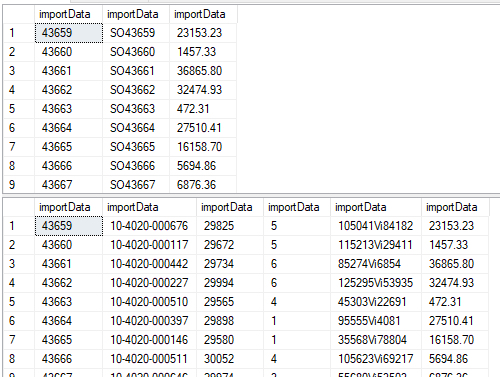As I said, I don’t know if there’s a better way to do this (there likely is, there are some clever folks out there), but this amused me for an afternoon so I thought I’d share in case it’s ever useful.

NB: Comments will only appear once they have been moderated.

## Kutech  CEOKevin  Urquhart